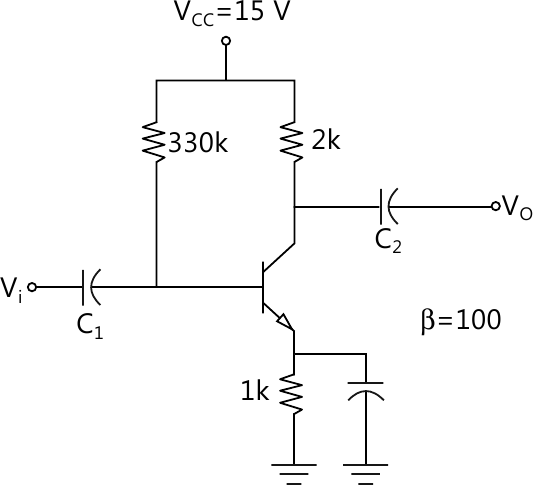MORE IN Analog and Digital Electronics
VTU Computer Science (Semester 3)
Analog and Digital Electronics
December 2012
Total marks: --
Total time: --
INSTRUCTIONS
(1) Assume appropriate data and state your reasons
(2) Marks are given to the right of every question
(3) Draw neat diagrams wherever necessary

1 (a) Explain transistor in its fixed bias mode with relevant expression.
6 M
1 (b) With a neat sketch, explain transistor as a switch.
6 M
1 (c) For the circuit shown calculate IB, IC, VCE, VC, VE, VB. Assume β=100.8 M

2 (a) Explain the VI characteristics of n-channel JFET and define various conditions.
8 M
2 (b) Explain the construction and working of n-channel depletion mode MOSFET.
8 M
2 (c) Mention merits and demerits of IGBT.
4 M

3 (a) Explain the construction and working of phototransistor and mention its applications.
10 M
3 (b) What are optocouplers? Explain the working and characteristics of optocoupler.
10 M

4 (a) Derive expression for Ai, Zi, Av, Yo, Ap for a transistor amplifier using h-parameter model.
12 M
4 (b) Explain the need for cascading amplifier and with the block diagram, explain two stage cascaded amplifier.
8 M

5 (a) Explain different fb amplifier.
8 M
5 (b) with the block diagram, explain the negative feedback in small amplifier.
6 M
5 (c) An amplifier having a voltage gain of 60dB uses 1/20th of its output in negative feedback. Calculate the gain with feednack, the percentage change in gain without and with feedback consequent on 50% change in gm.
6 M

6 (a) Explain the construction working of RC phase shift oscillator.
8 M
6 (b) Find the frequency of the oscillations of a Colpitts oscillator having C1=150pF, C2=1.5 nF and L=50μH.
4 M
6 (c) With a circuit diagram, explain the working of RC low pass and RC high pass circuits.
8 M

7 (a) With a block diagram, explain the working of three terminal voltage regulators.
6 M
7 (b) Explain the construction and working of SMPS and mention different types of switching regulators.
8 M
7 (c) Define the terms load regulation, line regulation and output resistance for a voltage regulator.
6 M

8 (a) Briefly explain characteristics of an ideal op-amp and compare with practical op-amp.
10 M
8 (b) With relevant formulas, neat diagram and wave form explain op-amp Schmitt trigger.
10 M

More question papers from Analog and Digital Electronics